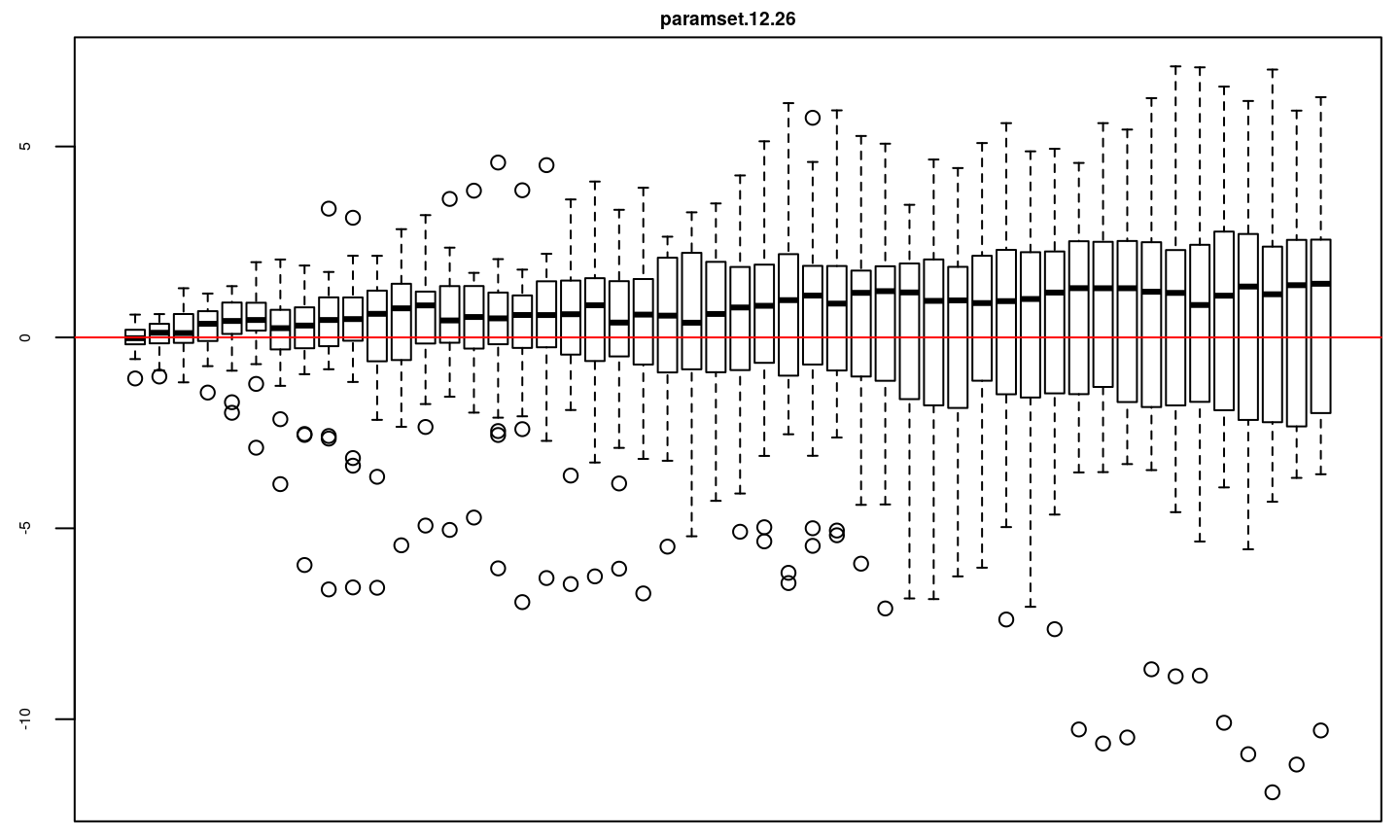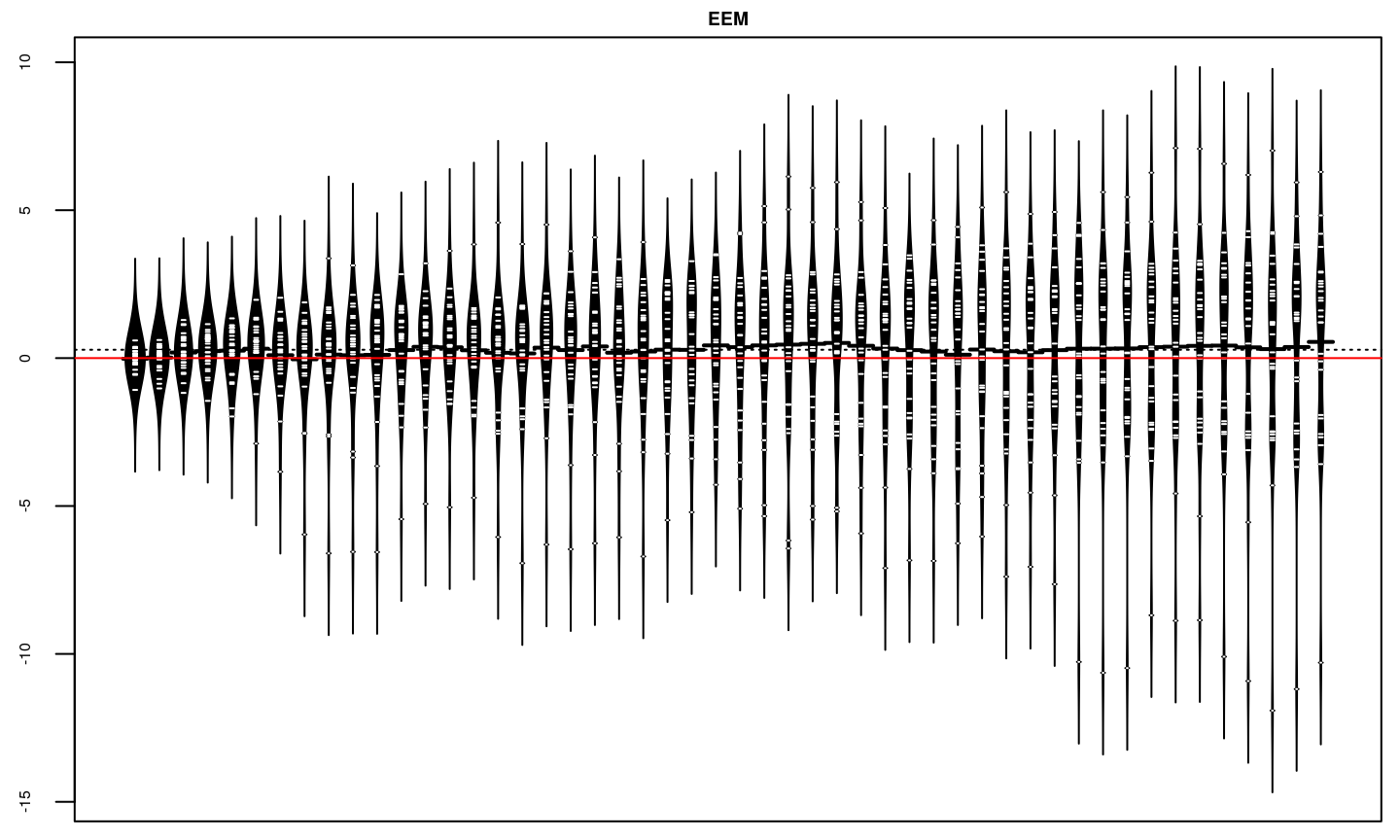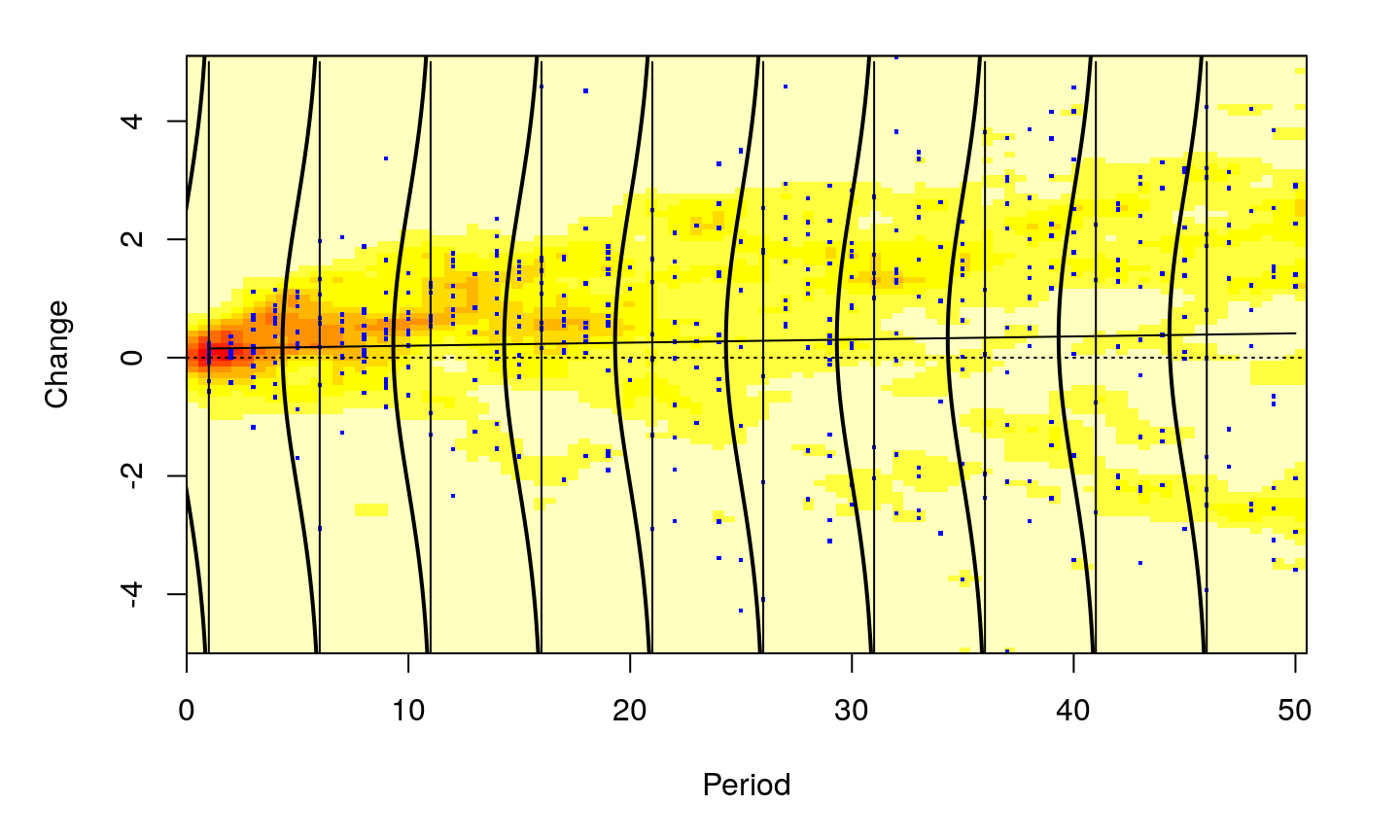compiled 2018-05-31

## Who is this Guy ?

Brian Peterson:

• quant, author, open source advocate
• author or co-author of over 10 packages for using R in Finance
• Lecturer, University Of Washington Computational Finance and Risk Management
• manage quant trading teams at several Chicago proprietary trading firms over time …

• proprietary or principal traders are a specific "member" structure with the exchanges
• high barriers to entry, large up-front capital requirements
• many strategies pursued in this structure have capacity constraints
• benefits on the other side are low fees, potentially high leverage
• money management needs to be very focused on drawdowns, leverage, volatility

## Backtesting, art or science?

Back-testing. I hate it - it's just optimizing over history. You never see a bad back-test. Ever. In any strategy. - Josh Diedesch (2014), CalSTRS

Every trading system is in some form an optimization. - Emilio Tomasini (2009)

## Moving Beyond Assumptions

Many system developers consider

"I hypothesize that this strategy idea will make money"

• build a hypothesis for the system
• build the system in pieces
• test the system in pieces
• measure how likely it is that you have overfit

## Constraints and Objectives

Constraints

• capital available
• execution platform

Benchmarks

• published or synthetic?
• what are the limitations?
• are you held to it, or just measured against it?

Objectives

• formulate objectives for testability

## Building a Hypothesis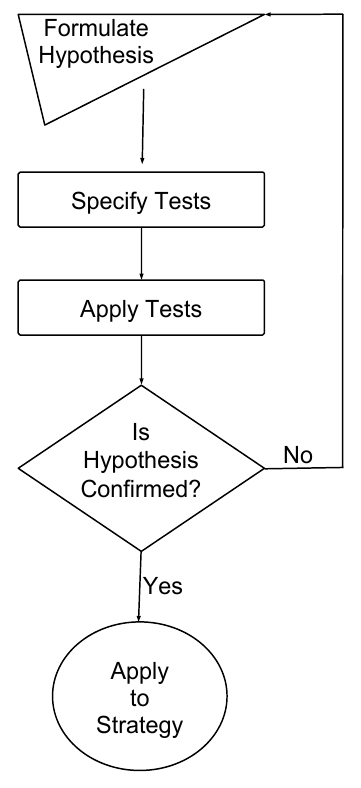To create a testable idea (a hypothesis):

• formulate a declarative conjecture
• make sure the conjecture is predictive
• define the expected outcome
• describe means of verifying/testing

good/complete Hypothesis Statements include:

• what is being analyzed (the subject),
• dependent variable(s) (the result/prediction)
• independent variables (inputs to the model)
• the anticipated possible outcomes, including direction or comparison
• addresses how you will validate or refute each hypothesis

## R in Finance trade simulation toolchain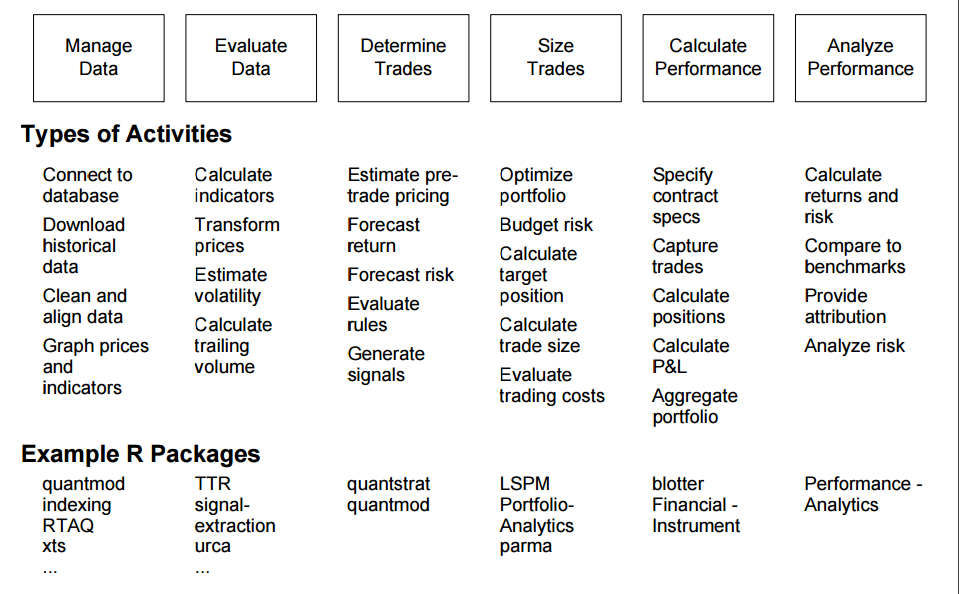## Building Blocks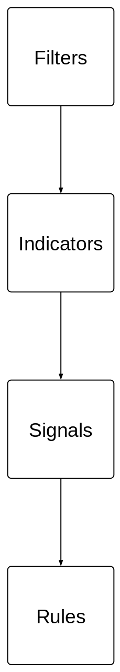Filters

• select the instruments to trade
• categorize market characteristics that are favorable to the strategy

Indicators

• values derived from market data
• includes all common "technicals"

Signals

• describe the interaction between filters, market data, and indicators
• can be viewed as a prediction at a point in time

Rules

• make path-dependent actionable decisions

## Installing blotter and quantstrat

• on Windows, you will need Rtools
```install.packages('devtools') # if you don't have it installed
install.packages('PerformanceAnalytics')
install.packages('FinancialInstrument')

devtools::install_github('braverock/blotter')
devtools::install_github('braverock/quantstrat')
```

## Our test strategy - MACD

```stock.str <- 'EEM'

currency('USD')
stock(stock.str,currency='USD',multiplier=1)

startDate='2003-12-31'
initEq=100000
portfolio.st='macd'
account.st='macd'

initPortf(portfolio.st,symbols=stock.str)
initAcct(account.st,portfolios=portfolio.st,initEq = initEq)
initOrders(portfolio=portfolio.st)

strategy.st<-portfolio.st
# define the strategy
strategy(strategy.st, store=TRUE)```
```## get data
getSymbols(stock.str,
from=startDate,
src='tiingo')```
• we'll use MACD as a simple trend follower for illustration
• I am not advocating MACD as an investment strategy
• we hypothesize that our MACD strategy will detect durable trends
• we also hypothesize that it will get chopped up by sideways markets because of the lag in the moving average

• don't pay a lot of attention to the code, this entire presentation is written using rmarkdown (Xie 2014), with references in BibTeX via JabRef and has been compiled for this presentation

## Test the System in Pieces

### How to Screw Up Less

Far better an approximate answer to the right question, which is often vague, than an exact answer to the wrong question, which can always be made precise. - John Tukey (1962) p. 13

Fail quickly, think deeply, or both?

No matter how beautiful your theory, no matter how clever you are or what your name is, if it disagrees with experiment, it’s wrong. - Richard P. Feynman (1965)

```#MA parameters for MACD
fastMA = 12
slowMA = 26
signalMA = 9
maType="EMA"

#one indicator
arguments = list(x=quote(Cl(mktdata)),
nFast=fastMA,
nSlow=slowMA),
label='_'
)```

MACD is a two moving average cross system that seeks to measure:

• the momentum of the change
• the divergence between the two moving averages

Classical technical analysis, for example only, not widely deployed in production

## Measuring Indicators

What do you think you're measuring? A good indicator measures something in the market:

• a theoretical "fair value" price, or
• the impact of a factor on that price, or
• turning points, direction, or slope of the series

Make sure the indicator is testable:

• hypothesis and tests for the indicator
• standard errors and goodness of fit
• t-tests or p-value
• goodness of fit
• custom 'perfect foresight' model built from a periodogram or signature plot

If your indicator doesn't have testable information content, throw it out and start over.

## Specifying tests for Indicators

• facts to support or refute

• general tests
• MSFE/MSFE
• confusion matrix
• standard errors
• Monte Carlo errors
• specific tests
• tests related to the model
• tests of the prediction
• tests of the relationships between variables

## General Diagnostics for Indicators

• Euclidean Distance
• often squared
• or de-meaned
• or lagged to line things up
• clustering models
• piece-wise linear decomposition
• mean squared forecast error

```#two signals
name="sigThreshold",
arguments = list(column="signal._",
relationship="gt",
threshold=0,
cross=TRUE),
label="signal.gt.zero"
)

name="sigThreshold",
arguments = list(column="signal._",
relationship="lt",
threshold=0,
cross=TRUE),
label="signal.lt.zero"
)```

## Combining Signals

Signals are often combined:

"\(A\) & \(B\)" should both be true.

This is a composite signal, and serves to reduce the dimensionality of the decision space.

A lower dimensioned space is easier to measure, but is at higher risk of overfitting.

Avoid overfitting while combining signals by making sure that your process has a strong economic or theoretical basis before writing code or running tests

## Measuring Signals

Signals make predictions so all the literature on forecasting is applicable:

• mean squared forecast error, BIC, etc.
• box plots or additive models for forward expectations
• "revealed performance" approach of Racine and Parmeter (2012)
• re-evaluate assumptions about the method of action of the strategy
• detect information bias or luck before moving on
```add.distribution(strategy.st,
paramset.label = 'signal_analysis',
component.type = 'indicator',
component.label = '_',
variable = list(n = fastMA),
label = 'nFAST'
)
#>  "macd"

paramset.label = 'signal_analysis',
component.type = 'indicator',
component.label = '_',
variable = list(n = slowMA),
label = 'nSLOW'
)
#>  "macd"```

## Run Signal Analysis Study

```sa_buy <- apply.paramset.signal.analysis(
strategy.st,
paramset.label='signal_analysis',
portfolio.st=portfolio.st,
sigcol = 'signal.gt.zero',
sigval = 1,
on=NULL,
forward.days=50,
cum.sum=TRUE,
include.day.of.signal=FALSE,
obj.fun=signal.obj.slope,
decreasing=TRUE,
verbose=TRUE)
#> Applying Parameter Set:  12, 26```
```sa_sell <- apply.paramset.signal.analysis(
strategy.st,
paramset.label='signal_analysis',
portfolio.st=portfolio.st,
sigcol = 'signal.lt.zero',
sigval = 1,
on=NULL,
forward.days=10,
cum.sum=TRUE,
include.day.of.signal=FALSE,
obj.fun=signal.obj.slope,
decreasing=TRUE,
verbose=TRUE)
#> Applying Parameter Set:  12, 26```